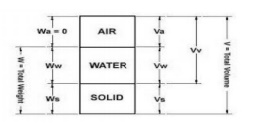Home | | Soil Mechanics | Soil Mechanics: Phases Relationship

# Soil Mechanics: Phases RelationshipThe three constituen ts are blended together to form a complex material. It is also known as block diagram. D ry soil and saturated soil is known as two ph ase diagram.

Soil Mechanics: Phases Relationship

Phases Relationship

The three constituen ts are blended together to form a complex material. It is also known as block diagram. D ry soil and saturated soil is known as two ph ase diagram.

Weight

Wt = Ww + Ws + Wg

Volume

Vt = Vv + Vs = Va + Vw + Vs + Vg

1. Volumetric Relation ships

i) Void ratio

It is defined as the ratio of volume of voids to the volume of soli ds.

e = Vv/Vs; the void ratio is expressed in decimal.

ii) Porosity (n) or Percentage of voids

It is defined as the ratio of the volume of voids to the total volum e.

n = Vv/V; Porosity is expre ssed as percentage & it is not exceed 100%.

n = e/1+e; e = n/n-1

iii) Degree of Saturation (S)

The degree of saturation (s) is the ratio of the volume of water to the volume of

voids.

S = Vw/Vv; The degree of saturation is generally as a percentage . It is equal to zero when the soil is absolutely dry & 100 % when the soil is fully saturated.

iv) Percentage of air voids (na)

It is defined a s the ratio of the volume of air to the total v olum, .na = Va/V (it is represented as(%)

v) Air Content (ac)

It is defined as the ratio of the volume of air to the volume of voids, .ac = Va/Ve,

Air Content is usually expressed as percentage. Air Content and percentage of air voids are zero when the soil is saturated.(Va=0)

na = Va/V = Va/Vv .Vv/V

na = n.ac

vi) Water content (w)

The water content (w) is defined as the ratio of the mass of water to mass of solids.

w = Mw/Ms. It is also known as moisture content (m); it is expressed as percentage but used as a decimal computation.

2. Volume Mass Relationships

i) Bulk density (?)

The bulk mass density (?) is defined as the total mass (m) per unit total volume (v)

? = m/v.It is also known as Bulk mass density, Bulk density, Wet mass density and density.

It is expressed as kg/m3 , gm/ml (or) mg/m3

ii) Dry mass density (?d)

The dry density (?d) is defined as the mass of solids per unit total volume.

?d =Ms/V

iii)Saturated density(?sat)

Saturated density the bulk mass density of the soil when it is fully saturated.

?sat=Msat/V

iv)Submerged density

When the soil exists below water it is submerged conditions. When a volume of v of soil is submerged in water, it displaces an equal volume of water.

v)Density of solids

Density of solids is equal to the ratio of the mass of solids to the volume of solids.

Rs=Ws/Vs

3. Volume-Weight Relationships

i)Bulk unit weight (?)

Bulk unit weight is defined as total weight per unit total volume.

? =W/V

ii) Dry unit weight (?d)

It is defined as the weight of soil solids per unit total volume. ?d= Ws/V

iii) Saturated unit weight

The saturated unit weight is bulk unit weight when the soil is fully saturated. It is defined as weight of saturated soil solids to the unit total volume. ?sat=Wsat/V

iv) Submerged unit weight (?')

It is defined as the submerged weight per unit of total volume.

?? = Wsub/V; ?sat = Wsub-?w

v) Unit weight of soil solids (?s)

The unit weight of solids (?s) is equal to the ratio of the weight of solids to the total volume of solids. = ?s= Ws/Vs

4 Specific gravity of solids (G)

i) The specific gravity of solid particles is defined as the ratio of the mass of a given volume of solids to the mass of an equal volume of water @ 4 o C. = G =ps/pw

The specific gravity of solids for most natural soils is range of 2.65 to 2.80.

ii) Mass specific gravity (or) apparent specific gravity (or) Bulk specific gravity

It is defined as the ratio of the mass density of the soil to the mass density of water.

Gn=p/pw

iii) Absolute specific gravity (or) True specific gravity

If all the internal voids of the particles are exclude from the determination the true volume of solids, then the specific gravity is called as Absolute (or) True specific gravity.

Ga=(ps)/pw

5. Density Index (ID)

Relative compactness of natural soil.

It is varies from 0 to 1

ID= emax - e   /   emax-emin

. e max = voids ratio in loosest state ;e min = voids ratio in densest state.

e = natural voids ratio of deposits; When the natural state of cohesion less soil in the densest form e = e min, ID = 1.

Relative Density  Density Description

0-15  Very loose

15-35 Loose

35-65 Medium

65-85 Dense

85-100        Very denseStudy Material, Lecturing Notes, Assignment, Reference, Wiki description explanation, brief detail
Civil : Soil Mechanics : Soil Classification And Compaction : Soil Mechanics: Phases Relationship |